# Review: DRCN — Deeply-Recursive Convolutional Network (Super Resolution)

## Increase Recursive Depth Up to 16 Layers, Without Introducing New Parameters, Better Than SRCNN.

It has been a long time not reviewing papers related to super resolution. This time, DRCN (Deeply-Recursive Convolutional Network) is shortly reviewed. Indeed, the authors of DRCN are also the authors of VDSR. 20 layers of 3×3 convolutions with the same size and same number of filters in VDSR reminded them to have a recursive convolution here. They are both published in 2016 CVPR, and DRCN has obtained more than 200 citations. (Sik-Ho Tsang @ Medium)

### Outline

1. DRCN Basic Model
2. Recursive-Supervision & Skip Connection
3. Loss Function
4. Results

### 1. DRCN Basic Model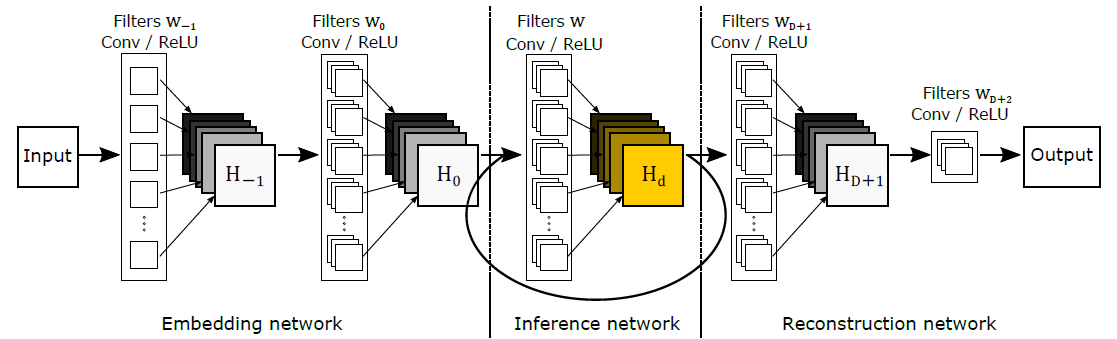DRCN Basic Model
• DRCN consists of three sub-networks: embedding, inference and reconstruction networks.

#### 1.1. Embedding Network: f1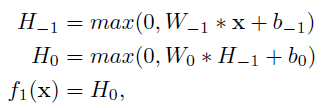Embedding Network
• It takes an interpolated low-resolution (LR) input image x (grayscale or RGB) and represents it as a set of feature maps H0 with two convolutions.

#### 1.2. Inference Network: f2

• The output feature maps from embedding network goes through a single recursive layer. Each recursion applies the same convolution followed by a ReLU.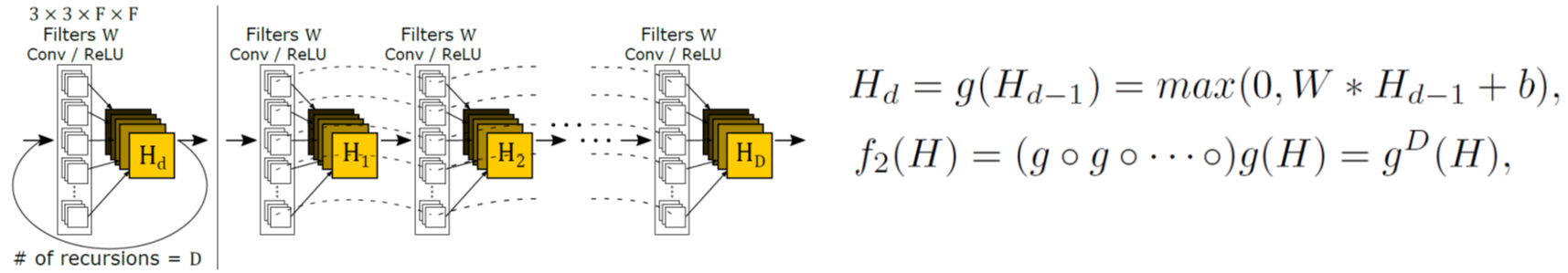Unfolding the Inference Network
• If we unfold the inference network, it looks like as above.
• D convolutions are performed with sharing parameters. Thus, there is no increase in number of parameters when more recursive layers are added.
• The receptive field is widened with every recursion.

#### 1.3. Reconstruction Network: f3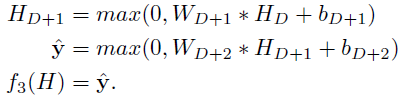Reconstruction Network
• It transforms the output feature maps from the inference network (multi-channel) into the original image space, i.e. high-resolution (HR) image.

#### 1.4. Pros and Cons

• The recursive model is simple and powerful.
• But training a deep-recursive network is very difficult due to vanishing gradients problem.

### 2. Recursive-Supervision & Skip Connection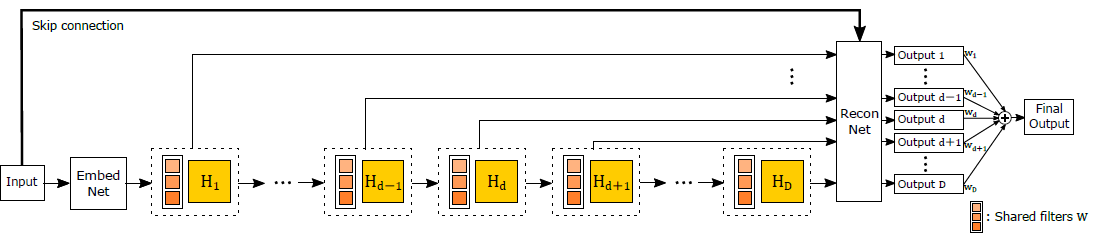DRCN with Recursive-Supervision & Skip Connection

#### 2.1. Recursive-Supervision

• As recursive convolution is used, we can pass the feature maps to reconstruction network at any recursion.
• In DRCN, for each recursion, the intermediate feature maps are also transmit to reconstruction network for reconstructing the HR image.
• Thus, there are in total D outputs as shown at the right above.
• An ensemble of all outputs with weighting significantly boost the performance.
• The adversarial effect of vanishing/exploding gradients along one backpropagation path is alleviated.
• The importance of picking the optimal number of recursions is reduced. If recursions are too deep for the given task, we expect the weight for late predictions to be low while early predictions receive high weights.

#### 2.2. Skip Connection

• Similar to VDSR, a skip connection is added as shown the figure above from input to reconstruction network.
• First, network capacity to store the input signal during recursions is saved.
• Second, the exact copy of input signal can be used during target prediction.
• In super-resolution, LR and HR images are vastly similar. In most regions, differences are zero and only small number of locations have non-zero values. This domain-specific knowledge significantly improves the learning procedure.
• Of course, it can address vanishing gradients issue.

### 3. Loss Function

• l1-loss: MSE between D outputs and the ground-truth HR image using recursive-supervision.
• l2-loss: MSE between the weighted D outputs and the ground-truth HR image.
• The final loss function: A weighted sum of l1-loss and l2-loss plus weight decay.

### 4. Results

• Training: 91 images, roughly takes 6 days using Titan X GPU.
• Testing: Four dataset of Set5, Set14, B100 and Urban100.

#### 4.1. Number of Recursions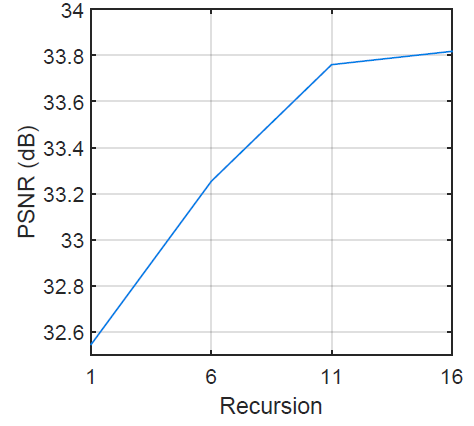Recursion versus Performance for the scale factor 3× on the dataset Set5
• 1, 6, 11, and 16 number of recursions are tested.
• More recursions yielding larger receptive fields and more non linearity lead to better performances. And 16 is chosen as optimum.
• When the 16-recursion DRCN is unfolded, the longest chain from the input to the output passes 20 convolution layers (receptive field of 41×41).

#### 4.2. Individual Output versus Ensembled Output

• There is no single recursion depth that works the best across all scale factors.
• Ensemble of intermediate predictions significantly improves performance.

#### 4.3. Comparison with state-of-the-art Approaches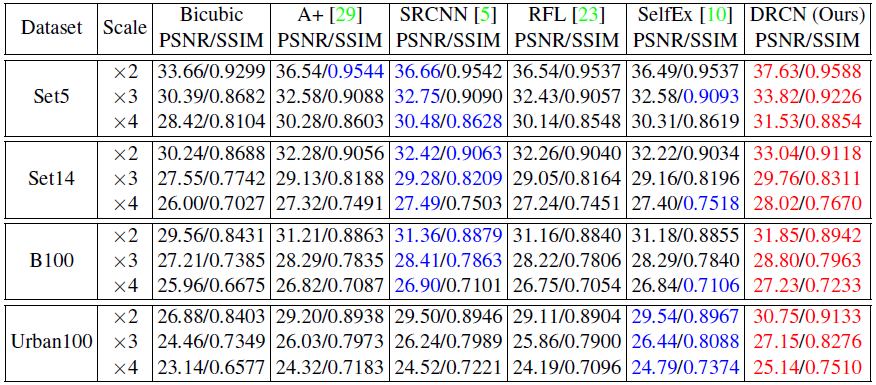Benchmark Results
• Since A+ and RFL use the center part only for evaluation but not image boundary. The above PSNR and SSIM are measured using the center part only.
• DRCN outperforms SRCNN for all scaling factors and datasets.

#### 4.4. Qualitative Results

By using recursion, more layers can be added without adding any extra parameters can be achieved, and the results are improved.